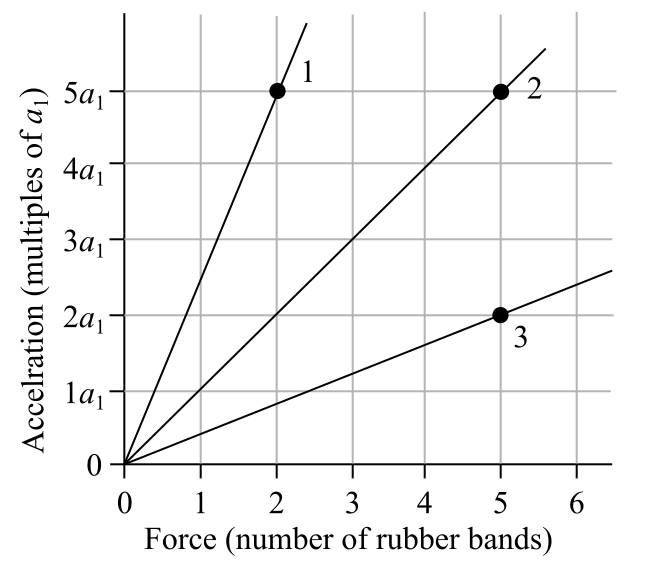# Problem: The figure shows an acceleration-versus-force graph for three objects pulled by rubber bands. The mass of object 2 is 0.30 kg.What is the mass of object 3?

###### FREE Expert Solution

Newton's second law:

$\overline{){\mathbf{\Sigma }}{\mathbf{F}}{\mathbf{=}}{\mathbf{m}}{\mathbf{a}}}$

From object 2, a1 = 3.33 m/s2

Solving for mass:

m = F/a

95% (335 ratings)###### Problem Details

The figure shows an acceleration-versus-force graph for three objects pulled by rubber bands. The mass of object 2 is 0.30 kg.

What is the mass of object 3?